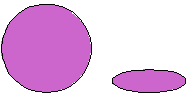Email us to get an instant 20% discount on highly effective K-12 Math & English kwizNET Programs!

#### Online Quiz (WorksheetABCD)

Questions Per Quiz = 2 4 6 8 10

### Grade 4 - Mathematics12.14 Geometry Part Three - Review Test

 Q 1: What is the area of a rectangle with length 6 inches and width 4 inches24 sq inches10 sq inches24 sq cms Q 2: The perimeter isthe distance around the figurehow much space it occupiesthe space inside a figure Q 3: In the figure shown are the three circles similarYesNo Q 4: A device for locating points in a plane is called ____. It is formed by drawing two number lines at right angles to each other and intersecting at their zero points.ordered number paircoordinatescoordinate grid Q 5: Are the two triangles in the figure congruent? (hint: sometimes you have to turn the figure around to see if it is congruent with another)yesno Q 6: Perimeter of a rectangle P = 2 x (l + w) can also be written asP = l + l + w + wP = l + 2wP = w + 2l Q 7: Are these similar figures?NoYes Q 8: A sphere hasinfinite edges2 edge0 edge1 edge Question 9: This question is available to subscribers only! Question 10: This question is available to subscribers only!